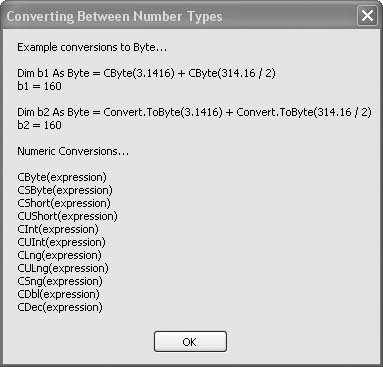# Recipe 6.7. Converting Between Number Types

#### Problem

You want to explicitly convert numeric variables and calculation results between the various number types.

#### Solution

Sample code folder: Chapter 06\ConvertNumber

It's always a good idea to make sure your project's Option Explicit and Option Strict settings are on, but this often forces you to apply explicit conversions when working with more than one type of numeric variable. The solution is to apply one of the many standalone conversion functions provided by Visual Basic or to use one of the many methods of the Convert object.

#### Discussion

The following code sample demonstrates a simple conversion of Double numeric values to Byte values, using both the standalone CByte() function and the Convert.ToByte() method. Some people prefer to use the Convert object exclusively, which may be easier to remember because all the conversion methods have names beginning with "To". Others prefer the standalone conversion functions, because many of these have been around in previous versions of Visual Basic for some time now. We look at both approaches here:

` Dim result As New System.Text.StringBuilder Dim b1 As Byte = CByte(3.1416) + CByte(314.16 / 2) Dim b2 As Byte = Convert.ToByte(3.1416) + _    Convert.ToByte(314.16 / 2) result.AppendLine("Example conversions to Byte…") result.AppendLine() result.AppendLine("Dim b1 As Byte = CByte(3.1416) + " & _    "CByte(314.16 / 2)") result.Append("b1 = ") result.AppendLine(b1.ToString) result.AppendLine() result.Append("Dim b2 As Byte = Convert.ToByte(3.1416) + ") result.AppendLine("Convert.ToByte(314.16 / 2)") result.Append("b2 = ") result.AppendLine(b2.ToString) result.AppendLine() result.AppendLine("Numeric Conversions…") result.AppendLine() result.AppendLine("CByte(expression)") result.AppendLine("CSByte(expression)") result.AppendLine("CShort(expression)") result.AppendLine("CUShort(expression)") result.AppendLine("CInt(expression)") result.AppendLine("CUInt(expression)") result.AppendLine("CLng(expression)") result.AppendLine("CULng(expression)") result.AppendLine("CSng(expression)") result.AppendLine("CDbl(expression)") result.AppendLine("CDec(expression)") MsgBox(result.ToString()) `

The Double value 314.16 will not convert to a Byte because it is out of range for byte values. Attempting this conversion causes an exception. However, dividing this value by 2 results in a Double value that does convert. The point is that the decimal digits don't cause a problem when converting to a Byte (they are simply rounded to the nearest byte value), but the number must be in the range 0 to 255 to allow the conversion.

Figure 6-7 shows the results of the above demonstration code in action. A sample conversion is shown using both techniques, and a list of the standalone conversion functions is displayed for easy review.

##### Figure 6-7. Different ways of converting between number typesThe signed byte and unsigned integer data types are new with this latest version of Visual Basic, and so are the functions to convert values to them.

See "conversion functions" in Visual Studio Help for more information on these functions.Visual Basic 2005 Cookbook: Solutions for VB 2005 Programmers (Cookbooks (OReilly))
ISBN: 0596101775
EAN: 2147483647
Year: 2006
Pages: 400

Similar book on Amazon